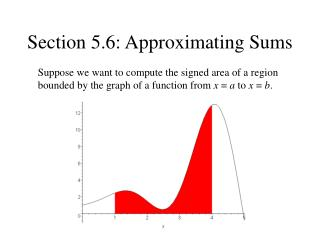DownloadDownload PresentationSection 5.6: Approximating Sums

# Section 5.6: Approximating Sums

Download Presentation## Section 5.6: Approximating Sums

- - - - - - - - - - - - - - - - - - - - - - - - - - - E N D - - - - - - - - - - - - - - - - - - - - - - - - - - -
##### Presentation Transcript

1. Section 5.6: Approximating Sums Suppose we want to compute the signed area of a region bounded by the graph of a function from x = a to x = b.

2. Let’s try an easier one first...

3. Now, back to the bigger challenge... Maybe we can approximate the area...

4. Maybe we can approximate the area... An underestimate!

5. Maybe we can approximate the area... An overestimate!

6. Maybe we can approximate the area... Looking better!

7. Maybe we can approximate the area... Better yet!

8. Definition of a Riemann Sum Let the interval [a, b] be partitioned into n subintervals by any n+1 points a = x0 < x1 < x2 < … < xn-1 < xn = b and let xi = xi – xi-1 denote the width of the ith subinterval. Within each subinterval [xi-1, xi], choose any sampling point ci. The sum Sn = f (c1)x1 + f (c2)x2 + … + f (cn)xn is a Riemann sum with n subdivisions for f on [a, b].

9. Commonly Used Riemann Sums • Left-hand • Right-hand • Midpoint

10. The Definite Integral as a Limit Let a function f (x) be defined on the interval [a, b]. The integral of f over [a, b], denoted is the number, if one exists, to which all Riemann sums Sn tend as as n tends to infinity and the widths of all subdivisions tend to zero. In symbols:

11. Trapezoid Rule

12. Trapping the Integral, Part I • Suppose f is monotone on [a, b]. Then, for any positive integer n, • If f is increasing, • If f is decreasing,

13. Trapping the Integral, Part II • For any positive integer n, • If f is concave up on [a, b], • If f is concave down on [a, b],

14. Simpson’s Rule For any positive integer n, the quantity is the Simpson’s rule approximation with 2n subdivisions.